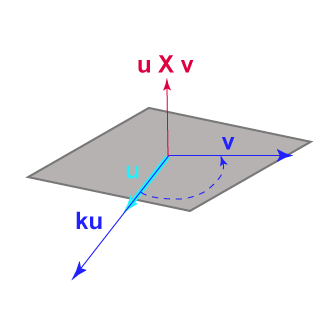Is the result of u × u   =   0   perpendicular to both operands?

Yes, because 0 · u   =   0, which means that they are perpendicular.

(Recall that by definition, the vector 0 is perpendicular to every vector.)

# More Math FunWhat about u × 0 ? The magnitude |u||0| sin θ is zero, and the result is a vector, so:

u × 0 = 0 × u = 0

Now, consider (ku) × v. Plug into the formula for the magnitude, |u||v| sin θ, see what you get.

### QUESTION 8:

What is (ku) × v ?